• 累计撰写 164 篇文章
• 累计创建 25 个标签
• 累计收到 208 条评论

### 目 录CONTENT# 实现图的遍历2020-07-28 / 0 评论 / 1 点赞 / 260 阅读 / 10,691 字

## 图遍历思想

• 深度优先搜索采用来存储待访问顶点
• 广度优先搜索采用队列来存储待访问顶点

• 白色：标识这个顶点还没被访问
• 灰色：标识这个顶点被访问过，但未被探索过
• 黑色：标识这个顶点被访问过且被完全探索过

## 广度优先搜索

### 实现思路

• 获取参数图(graph)的所有顶点和邻接表，将获取到的顶点初始化为白色，声明一个变量(color)接收初始化后的顶点对象
• 创建一个队列(queue)，将开始顶点(startVertex)入队
• 如果队列非空，则执行以下步骤
• 取出队列里存储的顶点u，获取u的邻接表
• 标识顶点u的颜色为灰色，即已访问但未被探索
• 遍历u的邻接表，取出邻接表中的每个顶点w，如果w未被访问则将其标识为灰色，将w加入队列。
• 当邻接表中所有的顶点都被标识为灰色后，标识u顶点已被完全探索，将其标识为黑色
• 如果参数回调函数(callback)存在，则执行回调函数

### 实现代码

/**
* 广度优先搜索
* @param graph 需要进行搜索的图
* @param startVertex 开始顶点
* @param callback 得到每个节点后的回调函数
*/
export const breadthFirstSearch = (graph: Graph, startVertex: string | number, callback: (val: string | number) => void): void => {
// 获取图的所有顶点
const vertices = graph.getVertices();
// 获取图的临接表
// 将顶点进行初始化
const color = initializeColor(vertices);
// 实例化一个队列
const queue = new Queue();
// 将开始顶点入队
queue.enqueue(startVertex);
// 如果队列不为空就继续执行
while (!queue.isEmpty()) {
// 取出队列里存储的顶点u
const u = queue.dequeue();
// 获取取出顶点的临接表
const neighbors = <(string | number)[]>adjList.get(u);
// 将顶点列表里的u标识为已被访问但未被探索
color[u] = Colors.GERY;
// 遍历当前取出顶点的临接表
for (let i = 0; i < neighbors.length; i++) {
// 获取临接表中的每个顶点w
const w = neighbors[i];
// 如果w没被访问过
if (color[w] === Colors.WHITE) {
// 标识w为已被访问但未被探索
color[w] = Colors.GERY;
// 将w加入队列
queue.enqueue(w);
}
}
// 此时u顶点与其相邻顶点已经被探索，将u标识为已被访问且被完全探索
color[u] = Colors.BLACK;
// 执行回调函数
if (callback) {
callback(u);
}
}
};


### 编写测试代码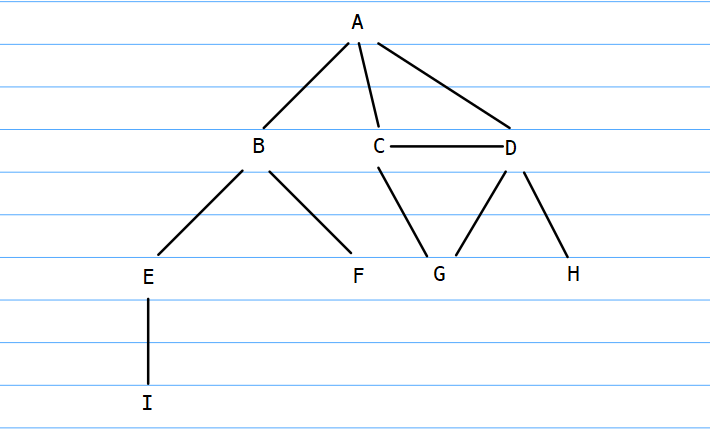• 构建图并建立顶点与顶点之间的关系
let graph = new Graph();
let vertices = ["A", "B", "C", "D", "E", "F", "G", "H", "I"];
for (let i = 0; i < vertices.length; i++) {
}

• 创建回调函数，执行广度优先搜索函数
const printVertices = (val) => {
console.log(val);
};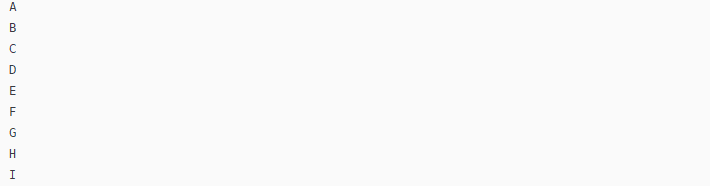### 求最短路径

• 从v到u的距离distances[u]
• 前溯点predecessors[u]，用来推导出从v到其他每个顶点u的最短路径

• 声明两个对象distancespredecessors分别用来存储距离和前溯点
• 遍历所有顶点，将每个顶点的距离初始化为0，将每个顶点的前溯点初始化为null
• 遍历邻接表时，发现顶点u的临点w时，通过给distances[u]的值+1来增加v和w之间的距离，设置w的前溯点值为u
• 最后，返回distances对象和predecessors对象

/**
* 广度优先搜索优化
* @param graph 要进行遍历的图
* @param startVertex 开始顶点
* @constructor
*/
export const BFS = (
graph: Graph,
startVertex: string | number
): { distances: { [key: string]: string | number }; predecessors: { [key: string]: string | number | null } } => {
// 获取图的所有顶点
const vertices = <(string | number)[]>graph.getVertices();
// 获取图的临接表
// 初始化顶点颜色
const color = initializeColor(vertices);
// 创建一个队列
const queue = new Queue();
// 存储每个顶点的距离
const distances: { [key: string]: string | number } = {};
// 存储前溯点
const predecessors: { [key: string]: string | null | number } = {};
// 顶点入队
queue.enqueue(startVertex);

// 遍历所有顶点
for (let i = 0; i < vertices.length; i++) {
// 用0来初始化每个顶点的距离
distances[vertices[i]] = 0;
// 用null来初始化每个顶点的前溯点
predecessors[vertices[i]] = null;
}

while (!queue.isEmpty()) {
// 获取队首顶点u
const u = queue.dequeue();
// 获取u的临接表
const neighbors = <(string | number)[]>adjList.get(u);
// u标识为已访问但未被探索状态
color[u] = Colors.GERY;
// 遍历u的临接表
for (let i = 0; i < neighbors.length; i++) {
// 获取临接表中遍历到的顶点w
const w = neighbors[i];
// 如果顶点w未被访问
if (color[w] === Colors.WHITE) {
// 标识顶点w为已访问但为被探索
color[w] = Colors.GERY;
// 给u顶点加1来增加v和w之间的距离（u是w的前溯点）
distances[w] = <number>distances[u] + 1;
// 发现顶点u的邻点w时，则设置w的前溯点值为u
predecessors[w] = u;
// w入栈
queue.enqueue(w);
}
}
}
return {
distances,
predecessors
};
};


const shortestPaths = BFS(graph, vertices);
console.log(shortestPaths);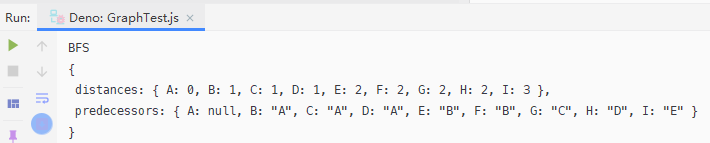# 距离
distances: { A: 0, B: 1, C: 1, D: 1, E: 2, F: 2, G: 2, H: 2, I: 3 }

# 前溯点
predecessors: { A: null, B: "A", C: "A", D: "A", E: "B", F: "B", G: "C", H: "D", I: "E" }
顶点A的前溯点为null
顶点B的前溯点为A
顶点C的前溯点为A
顶点D的前溯点为A
顶点E的前溯点为B
顶点F的前溯点为为B
顶点G的前溯点为C
顶点H的前溯点为D
顶点I的前溯点为E



• 遍历除过源顶点外的顶点列表
• 获取当前遍历到的顶点toVertex
• 创建一个栈，用于存储路径值
• 追溯toVertex到源顶点的路径，声明变量v默认值为toVertex，将其赋值为其前溯点的值
• 将v入栈
• 将源顶点入栈
• 声明变量s用于存储最短路径，依次取出栈中的元素，将其用-拼接
• 打印s
/**
通过前溯点列表获取顶点A到其他顶点的路径
*/
// 用顶点A作为源顶点
const fromVertex = vertices;
// 遍历除过源顶点外的顶点
for (let i = 1; i < vertices.length; i++) {
// 获取A抵达的顶点
const toVertex = vertices[i];
// 创建一个栈来存储路径值
const path = new Stack();
// 追溯toVertex到fromVertex的路径，变量v赋值为其前溯点的值
for (let v = toVertex; v !== fromVertex; v = shortestPaths.predecessors[v]) {
// v入栈
path.push(v);
}
// 源顶点入栈
path.push(fromVertex);
let s = path.pop();
while (!path.isEmpty()) {
s += " - " + path.pop();
}
console.log(s);
}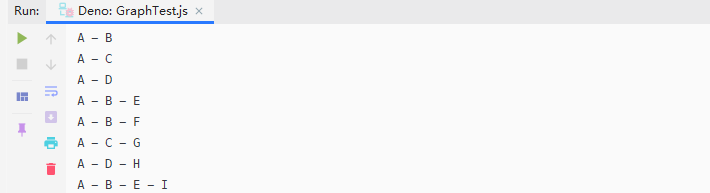## 深度优先搜索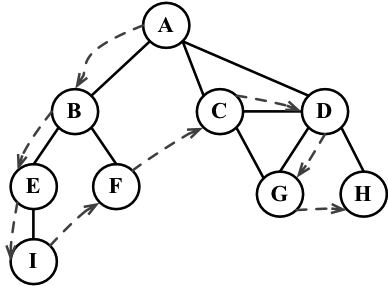### 实现思路

• 声明一个函数depthFirstSearch，该函数接收2个参数：要进行遍历的图、回调函数
• 获取图(graph)的顶点以及临接表，将获取到的顶点初始化为白色，用一个变量color来存储初始化后的顶点
• 遍历所有顶点，如果当前遍历到的顶点未被访问就递归访问其顶点

• 声明一个函数depthFirstSearchVisit，该函数接收4个参数：要访问的顶点、颜色对象、图的临接表、回调函数
• 首先，将要访问的顶点u标识为已发现状态
• 执行回调函数
• 获取u的临接表，遍历临接表
• 获取临接表的每个顶点w
• 如果w未被访问则以w为顶点继续进行递归访问
• 最后，遍历结束u已经被完全探索，将其标识为黑色。

### 实现代码

/**
* 深度优先搜索
* @param graph 要进行遍历的图
* @param callback 回调函数
*/
export const depthFirstSearch = (graph: Graph, callback: (val: string | number) => void): void => {
// 获取图的顶点
const vertices = graph.getVertices();
// 获取图的临接表
// 初始化顶点
const color = initializeColor(vertices);

for (let i = 0; i < vertices.length; i++) {
// 如果当前顶点未被访问
if (color[vertices[i]] === Colors.WHITE) {
// 调用递归函数进行递归访问
}
}
};

/**
* 递归访问顶点
* @param u 要访问的顶点
* @param color 颜色对象
* @param callback 回调函数
*/
const depthFirstSearchVisit = (
u: string | number,
color: { [p: string]: number },
adjList: Dictionary<string | number, (string | number)[]>,
callback: (val: string | number) => void
) => {
// 顶点u访问后，标识为已访问但未被探索状态
color[u] = Colors.GERY;
// 执行回调函数
if (callback) {
callback(u);
}
// 获取当前顶点的临接表
const neighbors = <string | number[]>adjList.get(u);
// 遍历临接表
for (let i = 0; i < neighbors.length; i++) {
// 获取临接表的每个顶点w
const w = neighbors[i];
// 如果w未被访问则以w为顶点进行递归访问
if (color[w] === Colors.WHITE) {
}
}
// u标识为已被完全探索
color[u] = Colors.BLACK;
};


### 编写测试代码

console.log("深度优先搜索节点访问顺序");
depthFirstSearch(graph, printVertices);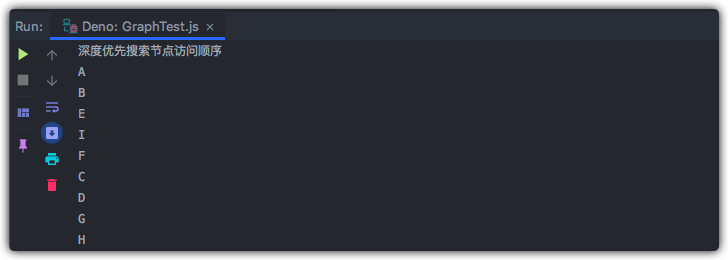### 拓扑排序

• 顶点u的发现时间d[u]
• 当顶点u被标注为黑色时，u的完成探索时间f[u]
• 顶点u的前溯点p[u]

• 声明3个对象d、f、p分别存储顶点的发现时间、完成探索时间、前溯点
• 声明time对象，用于记录每个顶点的访问时间
• 遍历顶点，将d、f、的每个顶点赋值为0，将p每个顶点的前溯点赋值为null

• 递归访问函数需要多传4个参数，d、f、p、time
• 当顶点被发现时，记录当前顶点u的初次发现时间，获取time的时间进行+1
• 当临接点w未被访问时存储w的前溯点
• 顶点u被完全访问后记录顶点的完成访问时间

/**
* 优化后的深度优先搜索
* @param graph 要进行搜索的图
* @constructor
*/
export const DFS = (
graph: Graph
): { predecessors: { [key: string]: string | number | null }; discovery: { [key: string]: string | number }; finished: { [key: string]: string | number } } => {
const vertices = <(number | string)[]>graph.getVertices();
const color = initializeColor(vertices);
const d: { [key: string]: string | number } = {};
const f: { [key: string]: string | number } = {};
const p: { [key: string]: string | number | null } = {};
const time: { [key: string]: number } = { count: 0 };
for (let i = 0; i < vertices.length; i++) {
f[vertices[i]] = 0;
d[vertices[i]] = 0;
p[vertices[i]] = null;
}
for (let i = 0; i < vertices.length; i++) {
if (color[vertices[i]] === Colors.WHITE) {
// 从i顶点开始递归访问
DFSVisit(vertices[i], color, d, f, p, time, adjList);
}
}

return {
discovery: d,
finished: f,
predecessors: p
};
};

/**
* 递归访问顶点
* @param u 要访问的顶点
* @param color 颜色对象
* @param d 顶点初次发现时间
* @param f 顶点完成访问时间
* @param p 前溯点
* @param time 初始时间
* @constructor
*/
const DFSVisit = (
u: string | number,
color: { [p: string]: number },
d: { [key: string]: string | number },
f: { [key: string]: string | number },
p: { [key: string]: string | number | null },
time: { [key: string]: number },
adjList: Dictionary<string | number, (string | number)[]>
) => {
// 顶点u被发现但未被探索
color[u] = Colors.GERY;
// 记录顶点u的初次发现时间
d[u] = ++time["count"];
// 获取顶点u的临接表
const neighbors = <(string | number)[]>adjList.get(u);
for (let i = 0; i < neighbors.length; i++) {
// 获取w临接点
const w = neighbors[i];
// 如果w未被访问，存储w的前溯点，以w未顶点继续递归访问
if (color[w] === Colors.WHITE) {
p[w] = u;
DFSVisit(w, color, d, f, p, time, adjList);
}
}
// 顶点被完全访问
color[u] = Colors.BLACK;
// 记录顶点完成访问时间
f[u] = ++time["count"];
};


console.log("优化后的深度优先搜索");
console.log(DFS(graph));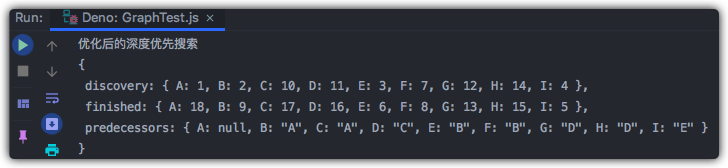顶点A发现时间是1，完成访问的时间是18

...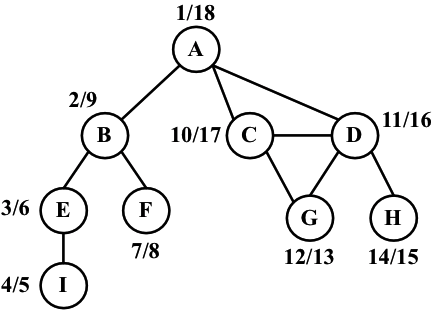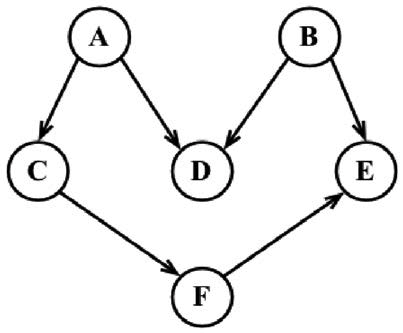• 构建一个有向图，将顶点依次加入图中
• 建立每个顶点之间的连接，执行优化后的深度优先搜索算法，获取其返回的数据result
• 获取result中的完成访问时间fTimes
• 声明变量s，用于存储拓扑排序最终的路径
• 遍历顶点列表
• 声明max和maxName
• 如果当前顶点的完成时间大于max就将max赋值为当前节点的完成时间，maxName赋值为当前遍历到的节点
• 拼接maxName
• 最后，删除fTimes中的max元素
• 打印拼接好的字符串s

// 实现拓扑排序
graph = new Graph(true);
vertices = ["A", "B", "C", "D", "E", "F"];
for (let i = 0; i < vertices.length; i++) {
}
const result = DFS(graph);
console.log("拓扑排序");
const fTimes = result.finished;
let s = "";
for (let count = 0; count < vertices.length; count++) {
let max = 0;
let maxName = null;
for (let i = 0; i < vertices.length; i++) {
if (fTimes[vertices[i]] > max) {
max = fTimes[vertices[i]];
maxName = vertices[i];
}
}
s += maxName + " - ";
delete fTimes[maxName];
}
console.log(s);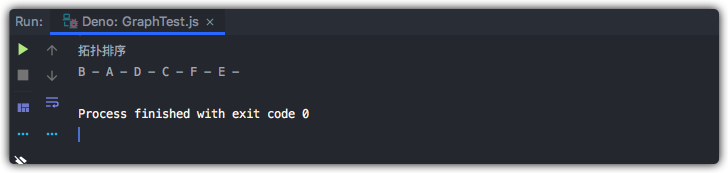## 写在最后

• 文中如有错误，欢迎在评论区指正，如果这篇文章帮到了你，欢迎点赞和关注😊
• 本文首发于掘金，未经许可禁止转载💌
1